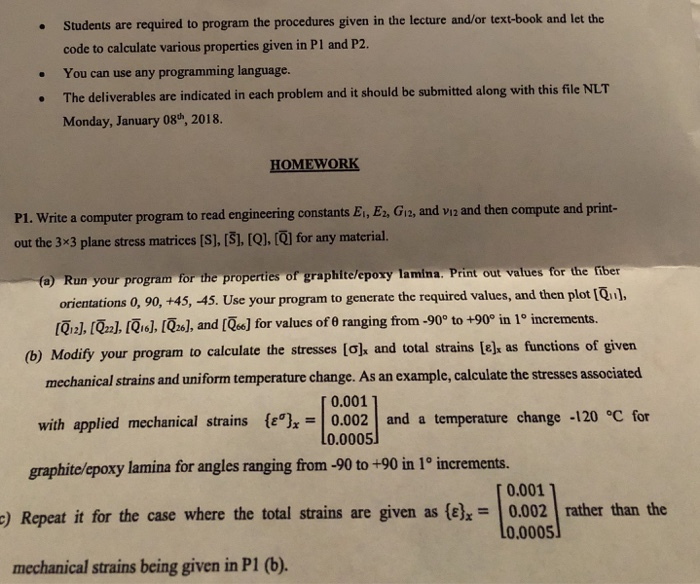Students are required to program the procedures given in the lecture and/or text-book and let the code to calculate various properties given in Pl and P2. You can use any programming language. The deliverables are indicated in each problem and it should be submitted along with this file NLT Monday, January 08, 2018. . HOMEWORK Write a computer program to read engineering constants Ei, E2, Gi2, and viz and then compute and print- out the 3×3 plane stress matrices [S], , [Q], [ for any material. fe) Run your program for the properties of graphite/epoxy lamina: Print out values for the fber orientations 0, 90, +45, -45. Use your program to generate the required values, and then plot [ [ 121, [on], [Qi。], [026,1, and [GJ (b) Modify your program to calculate the stresses [ ] for values of θ ranging from-90° to +90° in 1° increments. and total strains [has functions of given mechanical strains and uniform temperature change. As an example, calculate the stresses associated 0.001 with applied mechanical strains {ε%=10.0021 and a temperature change -120 °C for 0.0005 graphite/epoxy lamina for angles ranging from-90 to +90 in 1° increments. 0.001 Repeat it for the case where the total strains are given as (e),-10.0021 rather than the 0.0005 mechanical strains being given in P1 (b).